Courses

# Diode Rectifiers

## 10 Questions MCQ Test Topicwise Question Bank for GATE Electrical Engineering | Diode Rectifiers

Description
This mock test of Diode Rectifiers for Electrical Engineering (EE) helps you for every Electrical Engineering (EE) entrance exam. This contains 10 Multiple Choice Questions for Electrical Engineering (EE) Diode Rectifiers (mcq) to study with solutions a complete question bank. The solved questions answers in this Diode Rectifiers quiz give you a good mix of easy questions and tough questions. Electrical Engineering (EE) students definitely take this Diode Rectifiers exercise for a better result in the exam. You can find other Diode Rectifiers extra questions, long questions & short questions for Electrical Engineering (EE) on EduRev as well by searching above.
QUESTION: 1

### The circuit shown below is initially relaxed. With switch closed at t = 0, the conduction time of diode is around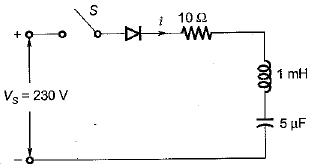Solution:

For the series RLC diode circuit, the current i(t) is given by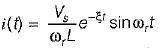Here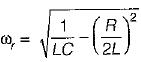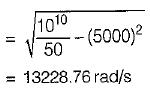The diode will stop conducting when ωr t1 = π.
∴ Conduction time of diode,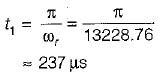QUESTION: 2

Solution:
QUESTION: 3

### A single-phase 230 V, 1 kW heater is connected across single-phase 230 V, 50 Hz supply through a diode as shown in figure. The power delivered to the heater element is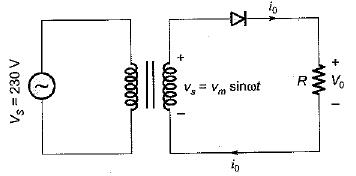Solution:

The rms value of output voltage is: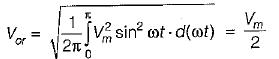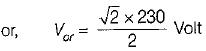Heater resistance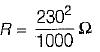∴ Power absorbed by heater element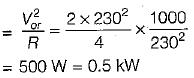QUESTION: 4

A single-phase full-bridge diode rectifier delivers a load current of 10 A, which is ripple free. The rms and average values of diode currents are respectively

Solution:

Given, I0 = 10A
The rms value of diode current,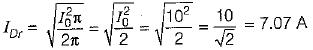The average value of diode current,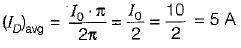QUESTION: 5

In the circuit shown below, the diode states at the extremely large negative value of the input voltage vi are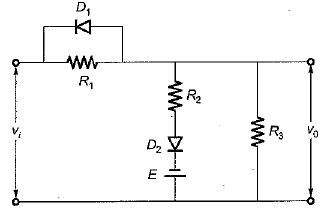Solution:

If vi is of very large negative value Ivi| > |E|, then diode D1 will be forward biased (ON) while diode D2 will be reverse biased (OFF).

QUESTION: 6

A single-phase full-bridge diode rectifier delivers a constant load current of 20 A. The rms and average values of source current are respectively

Solution:
QUESTION: 7

A diode whose internal resistance is 20 Q is to supply power to a 1 kΩ load from a 230 V (rms) source of supply as shown in figure below. The percentage regulation from no load to the given load is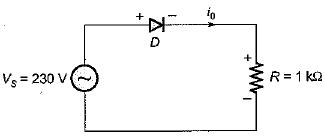Solution: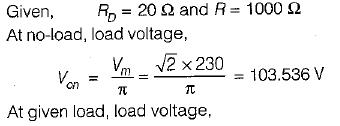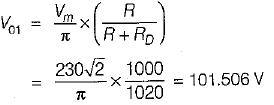∴ Voltage regulation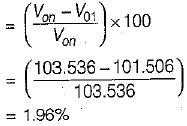QUESTION: 8

Assertion (A): A single-phase half-wave rectifier circuit is popularly known as one-pulse rectifier.
Reason (R) : A single-phase half-wave rectifier circuit is used to convert ac power to dc.

Solution:

A single-phase half-wave rectifier is popularly known as one-pulse converter because for one cycle of supply voltage, there is one half-cycle of output, or load voltage. Hence, both assertion and reason are true but reason is not the correct explanation of assertion.

QUESTION: 9

Assertion (A) : In 3-phase converters, the ripple frequency of the converter output voltage is higher than in single phase converter.
Reason (R) : The load current is mostly discontinuous in 3-phase converters.

Solution:

The load current is mostly continuous in 3-phase converters. Hence reason is a false statement.

QUESTION: 10

Assertion (A) : In a half-wave rectifier with R-L load and with freewheeling diode, the input Volt- Ampere demand is less.
Reason (R) : With freewheeling diode, the stored energy is in the load itself.

Solution: